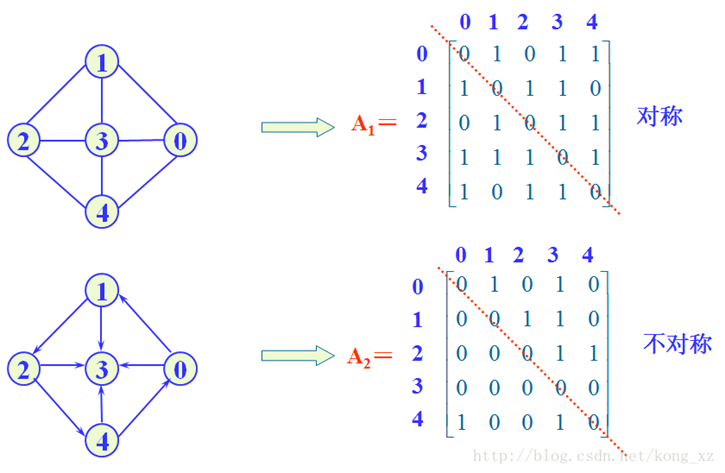• 题目说，对于一个有向图，请用邻接矩阵存储并且输出各个顶点出度和入度． 解题思路： 这题写出来就是为了好好学习下邻接矩阵写法，毕竟邻接矩阵也后续学习图论内容非常重要一个知识环节． 什么是了...
马上就开始去老校区进行数模培训了，听韩老师说，美赛很多题都是图论和网络流，于是打算近期恶补图论的相关知识了．
题目是说，对于一个有向图，请用邻接矩阵存储并且输出各个顶点的出度和入度．

解题思路：
这题写出来就是为了好好学习下邻接矩阵的写法，毕竟邻接矩阵也是后续学习图论内容非常重要的一个知识环节．
什么是了邻接矩阵呢？邻接矩阵说的就是对于一个图，把他的所有顶点都抽象出来成为Ｖ，把顶点与顶点间的关系抽象出来成为Ｅ，
那么不同顶点间肯定会存在边的关系，于是这样，我们就能把这样的一个图通过二维矩阵来描述出来了，如果Ｅｄｇｅ［ｉ］［ｊ］＝１，表示这两个顶点之间有边相互连接，
如果表示为０，表示这两个顶点之间没有边相互连接，好了，介绍完邻接矩阵后，再来看看顶点的出入度怎么求解了，我们知道，一个顶点的出入度可以分别从邻接矩阵中读出来了，究竟这个该怎么读呢？我们这样想，把第ｉ个顶点的这一行的值加起来，就对这应了该顶点的出度，把第ｉ个顶点的这一列的值加起来就对应了这个顶点的入度，那么这样一看的话，我们就能够很容易的计算出一个顶点的入度和出度了．．

代码：
# include<cstdio>
# include<iostream>
# include<cstring>

using namespace std;

# define MAX 100

int Edge[MAX][MAX];

int main(void)
{
int n,m;//顶点和边数
while ( cin>>n>>m )
{
int u,v;//边的起点和终点
int od,id;//顶点的入度和出度
if ( n == 0 && m == 0 )
{
break;
}
memset( Edge,0,sizeof(Edge) );
for ( int i = 1;i <= m;i++ )
{
cin>>u>>v;
Edge[u-1][v-1] = 1;
}
for ( int i = 0;i < n;i++ )
{
od = 0;
for ( int j = 0;j < m;j++ )
{
od += Edge[i][j];
}
if ( i==0 )
cout<<od;
else
cout<<" "<<od;

}
cout<<endl;

for ( int i = 0;i < n;i++ )
{
id = 0;
for ( int j = 0;j < n;j++ )
{
id += Edge[j][i];
}
if ( i == 0 )
cout<<id;
else
cout<<" "<<id;
}
cout<<endl;

}

return 0;
}

展开全文• 上部为无向，下部为有向 建图什么的不就有手！！就行！！ 使用实际或优劣对比： 1、方便检查任意一对定点间是否存在边 2、方便找任一顶点的所有“邻接点”（有边直接相连的顶点） 3、方便计算任一顶点的度(你遍历就...
图的几种存储结构：
1、邻接矩阵
2、链式前向星
3、C++中vector的邻接表
（一）邻接矩阵
邻接矩阵是表示顶点之间相邻关系的矩阵。
基本思想为：
S[i][j]就可以表示i ->（到） j有一条边内部数值可以是边权或者bool标记有无
上部为无向，下部为有向
建图什么的不就有手！！就行！！使用实际或优劣对比：
1、方便检查任意一对定点间是否存在边
2、方便找任一顶点的所有“邻接点”（有边直接相连的顶点）
3、方便计算任一顶点的度(你遍历就完了)
对于无向图，邻接矩阵的第i行（或第i列）非零元素（或非∞元素）的个数正好是第i个顶点的度。
对于有向图，邻接矩阵的第i行（或第i列）非零元素（或非∞元素）的个数正好是第i个顶点的出度（或入度）。
for(int i=1;i<=?;i++)if(a[i][j]!=0)du[i]++;

那么劣势是啥子嘞？？？时空关系恐惧症！！！！
（二）链式前向星
链表的话就是可以放很多东西嘛，理解的话稍微难度大一点
1、结构安排
struct edge{
int to;//  边是有向的，指向一个点
int next; //   锚定一个结点上的所有边，这个最难理解
int w;  //边权，可有可无
};

2、增边
int head[MAXN];  //记录这个点上现有最后一条边的num
int cnt;//全局，记录边的序号和个数，对于边的锚定也很重要
void  add(int from ,int to ，int w)
{
e[++cnt].next=head[from];
e[cnt].to=to;
e[cnt].w =w;
head[from]=cnt;
}

此处我们cnt=0，必须先自增；
3、锚定（这个蛮难的，姬某断断续续搞的差不多，真的绝绝子）
head[cnt]
记录了当前的节点的最后一条边，也是遍历的起点
每个节点第一边next=0；第二边指向第一边————最后head[点序号]指向第N边，N找到N-1(如此往复）最终到1；
（三）vector
vector就是（一）和（二）的结合，会有压边那种struct进去的直接二维vector
简单来一下二维vector就是动态了一下子，思想没变的,把from抽离出来就好了；
1、建立
struct edge{
int to;//  边是有向的，指向一个点
int w;  //边权，可有可无
}e;

vector<edge>vec[MAXN];

void  add(int from ,int to ,int w)
{
e.to=to;e.w=w;  //借助中间变量暂时存一下
vec[from].push_back(e);  //从from出发的边压了一条进去;
}


2、遍历
for(int i=1;i<=节点数;++i)
{
for (int j=0;j<vec[i].size();++j){
e=vec[i][j];  //借助中间量抽出我们的目的
cout<<"从 "<<i<<" 到 "<<e.to<<" 的值为 "<<e.value<<endl;
}
}


差不多就是这样的，姬某学了可能2周，初学者建议不要紧张，慢慢来就好了
爱与Oi系列


展开全文• 有向图卷积网络（Directed Graph Convolutional Network），源于2020年论文"Directed Graph Convolutional Network"；初次了解会给人一种感觉：这可能就是在GCN上小修改，但其实背后暗藏重大创新，在2018年出现...
目录有向图卷积网络简介Directed Graph Convolutional NetworkDiscuss GNN
有向图卷积网络简介
有向图卷积网络（Directed Graph Convolutional Network），简称DGCN，源于2020年的论文"Directed Graph Convolutional Network"；初次了解会给人一种感觉：这可能就是在GCN上的小修改，但其实背后暗藏重大创新，在2018年出现GCN前，我们就已经知道，Graph分为有向与无向，不管是什么样的图，总能用邻接矩阵表达，也能得到度矩阵，在该论文出现之前，我们其实完全可以认为用GCN就能胜任有向或无向图数据；但经过论文的描述，事实证明作者的想法确实有所道理；
比如现在有一个有向图：首先可以看出，边具备方向，另外，边具有粗细，这是带有权重的边；如果简单思考，这应该可以用图注意力网络GAT来做，可以对边施加注意力；
回忆GraphSAGE中的一阶邻居，二阶邻居，现在要补充一个新概念：一阶邻近，二阶邻近；比如节点1和节点3称为一阶邻近，节点1和节点2称为二阶邻近（因为它们共享节点4，5，6）；所以论文认为，对于有向图，不应当只考虑邻居，还要考虑邻近；

从邻近研究，有以下图：图a为原图，四个图中，以节点1作为主要研究对象，图b中节点4是节点1的一阶邻近，图c.i与图c.ii都是描述二阶邻近，对于图c.i，节点1和节点2是二阶邻近，对于图c.ii，节点1和节点3也是二阶邻近，但不同之处在于节点1和节点2称为二阶入度邻近，节点1和节点3称为二阶出度邻近；
Directed Graph Convolutional Network
针对有向图的一阶邻近表达，论文提出一阶邻近矩阵$A_{F}$：
$A_{F}(i,j)=A^{sym}(i,j)$
其中，$A^{sym}$是邻接矩阵$A$的对称形式，在有向图中，$A^{sym}(i,j)$元素取值规则为：

不存在一条边从节点$v_{i}$到节点$v_{j}$，或者从节点$v_{j}$到节点$v_{i}$，则$A^{sym}(i,j)=0$；
只要存在一条边从节点$v_{i}$到节点$v_{j}$，或者从节点$v_{j}$到节点$v_{i}$，则$A^{sym}(i,j)=1$；

注意：如果图是带权的，邻接矩阵$A$的元素将不局限与0和1，所以$A^{sym}$的值也不局限于0和1；

针对有向图的二阶邻近表达，论文提出了二阶入度邻近矩阵$A_{S_{in}}$，和二阶出度邻近矩阵$A_{S_{out}}$：
$A_{S_{in}}(i,j)=\sum_{k}\frac{A_{k,i}A_{k,j}}{\sum_{v}A_{k,v}},A_{S_{out}}(i,j)=\sum_{k}\frac{A_{i,k}A_{j,k}}{\sum_{v}A_{v,k}}$
其中，$A_{m,n}$表示原图邻接矩阵$(m,n)$处的元素；
对有向图分别进行三种变换：
$Z_{F}=\widetilde{D}_{F}^{-\frac{1}{2}}\widetilde{A}_{F}\widetilde{D}_{F}^{-\frac{1}{2}}X\Theta$
$Z_{S_{in}}=\widetilde{D}_{S_{in}}^{-\frac{1}{2}}\widetilde{A}_{S_{in}}\widetilde{D}_{S_{in}}^{-\frac{1}{2}}X\Theta$
$Z_{S_{out}}=\widetilde{D}_{S_{out}}^{-\frac{1}{2}}\widetilde{A}_{S_{out}}\widetilde{D}_{S_{out}}^{-\frac{1}{2}}X\Theta$
其中，$\Theta$为基于参数{$W,b$}的线性变换操作（即GCN在没有激活函数下的表达形式），和GCN一样，$\widetilde{A}_{x}=A_{x}+\lambda I$，$\widetilde{D}_{x}=D_{x}+\lambda I$，$x\in\left\{F,S_{in},S_{out}\right\}$；
对于邻近矩阵$A_{x}\in\mathbb{R}^{n\times n}$对应的度矩阵$D_{x}$，其中$x\in\left\{F,S_{in},S_{out}\right\}$，其计算规则为（与GCN中的度矩阵计算规则一样）：
$D_{x}(i,i)=\sum_{j}^{n}A_{x}(i,j)$

关于度矩阵的计算，不管是有向图，还是无向图，带权还是不带权，标准地，都可以通过邻接矩阵$A\in\mathbb{R}^{n\times n}$得到度矩阵$D$：
$D(i,i)=\sum_{j}^{n}A(i,j)$

Directed Graph Convolutional Network的架构为：综上，有向图卷积层模型$\widetilde{Y}=f(X,A)$为：
$\widetilde{Y}=Concat[ReLU(\widetilde{D}_{F}^{-\frac{1}{2}}\widetilde{A}_{F}\widetilde{D}_{F}^{-\frac{1}{2}}X\Theta^{(0)}),\alpha ReLU(\widetilde{D}_{S_{in}}^{-\frac{1}{2}}\widetilde{A}_{S_{in}}\widetilde{D}_{S_{in}}^{-\frac{1}{2}}X\Theta^{(0)}),\beta ReLU(\widetilde{D}_{S_{out}}^{-\frac{1}{2}}\widetilde{A}_{S_{out}}\widetilde{D}_{S_{out}}^{-\frac{1}{2}}X\Theta^{(0)})]$
其中，$\alpha$和$\beta$是可学习的参数，如果考虑对其进行全连接变换，再用softmax输出类别概率，模型延伸为：
$\widehat{Y}=softmax(ReLU(\widetilde{Y}\Theta^{(1)}))$
论文在Cora-ML数据集上对GCN和DGCN的表现进行了可视化：可视化的对象是单层图神经网络输出的特征，用非线性的降维方法将高维特征降维至二维，再将已知的节点类别标记成不同的颜色；
DGCN分为只考虑一阶邻近和一阶邻近二阶邻近都考虑的情况，从可视化结果中看，DGCN确实具备更强的特征变换能力，得到比GCN更简单的分布，从而有利于样本分类；
Discuss GNN
现在的GNN处于萌芽阶段，有很多方向等待人类探究，如果稍微观察，容易发现目前GNN的做法总是基于信息传递，信息聚合，信息更新三个步骤，也许研究如何让信息传递，聚合，更新变得更加合理和高效对于GNN是目前较为重要的工作；
GNN相比曾经热火多年的CNN，GNN的发展速度远没有CNN的发展迅猛，CNN从萌芽到爆发式增长也就经历了两三年时间，也许我们也应该考虑改变GNN的模式，从而获得CNN一样的迅速发展；
在大多数论文中，GNN总是在借鉴CNN的思想，而CNN专注于图像领域，GNN应该注重到其他更高级的领域，我们是否应该考虑打破借鉴的规则，让GNN具有专属于它的灵魂：展开全文人工智能 神经网络 机器学习
• ## 无向图的邻接矩阵

千次阅读 2016-05-30 11:28:30
1、图一种复杂的数据结构，表现在不仅各顶点的度可以不同，而且顶点之间的关系也错综复杂。从图的定义可知一个图的信息包括两个部分：图中顶点的信息和描述顶点之间关系——边或弧的信息。因此无论采取什么方法来...
1、图是一种复杂的数据结构，表现在不仅各顶点的度可以不同，而且顶点之间的关系也错综复杂。从图的定义可知一个图的信息包括两个部分：图中顶点的信息和描述顶点之间关系——边或弧的信息。因此无论采取什么方法来建立图的存储结构，都要完整、准确地反映这两部分的信息。
2、用邻接矩阵存储图，很容易确定图中任意两个顶点之间是否相连。但是，要确定图中具体有多少条边，则必须按行、按列对每个元素进行查找后方能确定，因此花费的时间代价较大，这也是用邻接矩阵存储图的局限性。

#include <stdio.h>
#include <stdlib.h>
#define MAXSIZE 20

typedef struct{
int vertex[MAXSIZE];            //节点信息
int edge[MAXSIZE][MAXSIZE];     //边信息
}Graph;

void createGraph(Graph * p,int n,int e){
Graph * g = p;

for(int i=0;i<n;i++){           //输入节点值
g->vertex[i] = i;

for(int j=0;j<n;j++){           //初始化邻接矩阵
for(int k=0;k<n;k++){
g->edge[j][k] = 0;
}
}

for(int t=0;t<e;t++){           //连通节点
printf("请输入边(i,j)\n");
scanf("%d,%d",&i,&j);
g->edge[i][j] = 1;
g->edge[j][i] = 1;
}
}

int main(){
Graph * p = (Graph *)malloc(sizeof(Graph));
int n,e;

printf("请输入节点的个数。\n");
scanf("%d",&n);
printf("请输入边数。\n");
scanf("%d",&e);
createGraph(p,n,e);
printf("无向图的邻接矩阵表示为：\n");

for(int i=0;i<n;i++){
for(int j=0;j<n;j++){
printf("%4d",p->edge[i][j]);
}
printf("\n");
}
return 0;
}
展开全文• 有向图中，一个结点经过两种路线到达另一个结点，未必形成环。 1 拓扑排序 1.1 无向图 使用拓扑排序可以判断一个无向图中是否存在环，具体步骤如下： 求出图中所有结点的度。 将所有度 <= 1 的结点入队。...算法 拓扑排序 dfs
• 应该注意的是顶点的度，顶点的度是连接一个顶点的边的数量，在有向图和无向图中顶点的度有很大的区别，在无向图中，顶点的度就是连接顶点边的数量，而在有向图中，由于线段具有方向性，顶点的度分为入度和出度，顶点...
• 邻接表：G[N]为指针数组，对应矩阵每行一个链表，只存非0元素 ... 对有向图：只能计算“出度”；需要构造“逆邻接表”(存指向自己边)来方便计算“入度” 方便检查任意一对顶点间是否存在边？No！ ...
• 无向图，有向图 ： 无向图A顶点度数3，有向图A出度1，入度2 根据边权值分类： 边无权值图，边有权值图 权值： 北京和大连之间权值7，北京和济南权值7 转载于:...
• ## 图的遍历

千次阅读 多人点赞 2017-03-11 12:58:08
这篇文章中总结一下关于图的遍历算法，在此之前，我们来看一下什么是图：首先，图可以分为有向图和无向图（这里只讨论无权图），像下面这个图就是无向图，V1 ~ V5 是图的顶点，而连接图的两个顶点的线就叫边或者专业...算法
• ## 图

2019-10-08 17:33:32
什么有向图，无向图，完全图 。。。感觉分那么清干嘛 度：顶点v的度是与它相关联的边的条数。 入度：是以v为终点的有向的条数出度：是以v为始点的有向边的条数 在有向图中顶点的度等于该顶点的入度与出度之和。 ...
• (a)有向图图的边有方向，只能按箭头方向从一点到另一点。 (b)无向图：图的边没有方向，可以双向。 基本概念： 结点的度：无向图中与结点相连的边的数目，称为结点的度。 结点的入度：在有向图中，以这个结点为...
• 图图的概念有向图和无向图形式化定义图度度的性质判断序列是否可图 图的概念 什么是图？ 图由一系列顶点和若干连结顶点集合内两个顶点的边组成的数据结构。 有向图和无向图 如果用边来表示好友关系，对于微信这种...算法
• 有向图的二分图做法不同，这个转化为求最少的欧拉路径。。 欧拉图有个结论欧拉路径的个数为为奇数的点的个数/2（可以类比欧拉回路的结论） 然后求欧拉路径的方法fleury算法。。其思想就是暴力dfs，然后...
• 分析：输入n个顶点，n-1条边，如果完全合法输入，那就简单的有向图，找入度为0节点，然后广搜或者深搜（深搜代码简单），得到最大深度就是树高度。 但是：只过了70%测试用例，我实在想不出来还有什么...
• 根据边是否有方向，可分为有向图和无向图。 2. 邻接点 两结点之间通过边相连，则互为邻接点。如在上面的无向图中，（1，3），（2，5）等都为邻接点。 3. 顶点的度 顶点的度指的与顶点v相连的边的数目。对于无...数据结构 最小生成树 普利姆算法
• 1.什么是顶点集？ 图中具有相同特性数据元素集合称为顶点集 2.什么是边（弧）？...在有向图中，每个顶点有两种，出度和入度。 （2）出度：顶点出度指从那个顶点出发数量。 （3）入度：顶点入度...数据结构
• 什么是图? 一邻接表表示法: 特点： 方便找任一顶点所有邻接顶点 ...有向图：只能计算出度 不方便检查任意一对顶点间是否存在边 #include<bits/stdc++.h> using namespace std; struct node{ int w;...数据结构
• DAG（有向无环计算机领域常用一种数据结构，它取消了“block”这个概念，进而也没有了“矿工”、“挖矿”这些。将组成单元从“block”变成一笔一笔“transaction”，如果用户想要发起一笔transaction，...区块链 比特币 linux ubuntu
• 根据边是否有方向，可分为有向图和无向图。 2. 邻接点 两结点之间通过边相连，则互为邻接点。如在上面的无向图中，（1，3），（2，5）等都为邻接点。 3. 顶点的度 顶点的度指的与顶点v相连的边的数目。对于无...邻接矩阵 数据结构
• 2.5.1 图是什么 图是由顶点(vertex,node)和边(edge)组成。顶点代表对象。在示意图中，我们使用点或圆来表示。边表示是两个对象连接关系。 无向图术语 两个顶点之间如果边连接，那么就视为两个顶点相邻。相邻...算法 链表 数据结构
• 行列式通常来讲一个数。而且这个数和面积（或者体积有关）。 例如，向量a和b行列式： 可以由a和b所张成平行四边形面积...a和b夹角应该90才对，为什么负面积呢？ 答案在于a和b给出顺序。 ...
• 图的术语（1）顶点（Vertex）（2）边（Edge）（3）有向图（4）无向图（5）有环图（6）无环图（7）（8）出度（9）入度4.图的经典表示法（邻接矩阵）二.Spark Graphx1.Spark GraphX 简介（1）Graphx是什么？（2）...spark 基本概念
• 在IE6,7下只要不设置Div高度，就能自适应高度，背景色或背景也能自动延伸。...这种方法浏览器兼容性好，没有什么问题，缺点就是需要额外（而且通常无语义）标签。  在Div末尾加入代码： 　＜di
• 一些简单工具可用来允许用户地图贡献自己标记或路线。 有关更多信息，请参见贡献。 该项目开源。 欢迎提出问题和要求，并高度赞赏。 为什么选择GenshinMap？ 还有许多其他交互式地图可用，但是此...
• IPFS作为区块链近十年以来唯一落地项目，随着这项技术一一个推广，会越来越多人需要通过购买fil币来我们矿工支付存储等相关费用，而fil币总量恒定，fil币作为唯一一个支付凭证， 那它未来价值也...区块链 比特币 ipfs 大数据 服务器
• 茂强）一个网络Network一个树Tree一个RDBMSRDMBMS一个稀疏矩阵稀疏矩阵网络或者Kitchensink顶点顶点边边graphx一个图计算引擎，而不是一个图数据库，它可以处理像倒排索引，推荐系统，最短路径，群体检测等等有向......